Homework Help Question & Answers

# Use the function to find the image of v and the preimage of w. T(V1, V2,...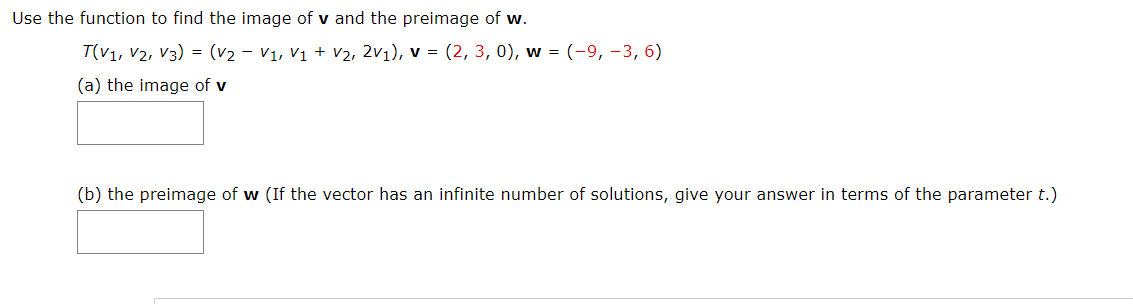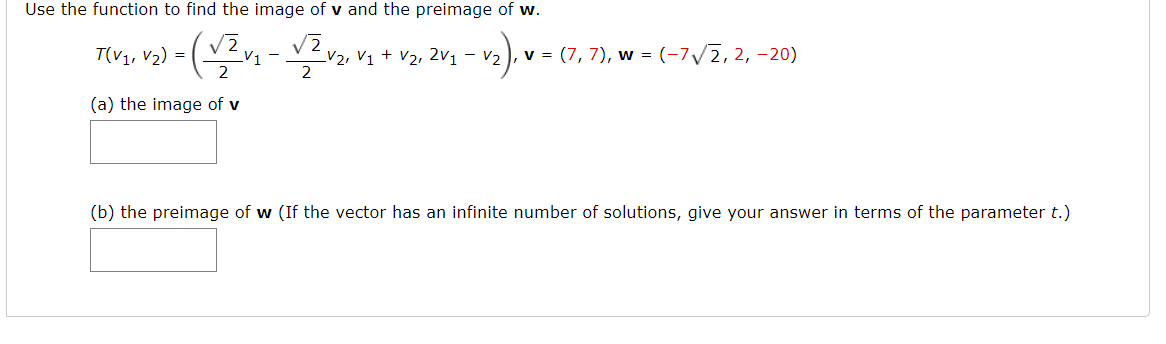Use the function to find the image of v and the preimage of w. T(V1, V2, V3) = (v2 - V1, V1 + V2, 2v1), v = (2,3,0), w = (-9, -3, 6) (a) the image of v (b) the preimage of w (If the vector has an infinite number of solutions, give your answer in terms of the parameter t.)
Use the function to find the image of v and the preimage of w. TIV3, va) = (v2v2 - y2 v2, va + v2, 2va – vz), v= (1,7), w = (-7/2, 2, -20) (a) the image of v (b) the preimage of w (If the vector has an infinite number of solutions, give your answer in terms of the parameter t.)

#### Homework Answers

Answer #1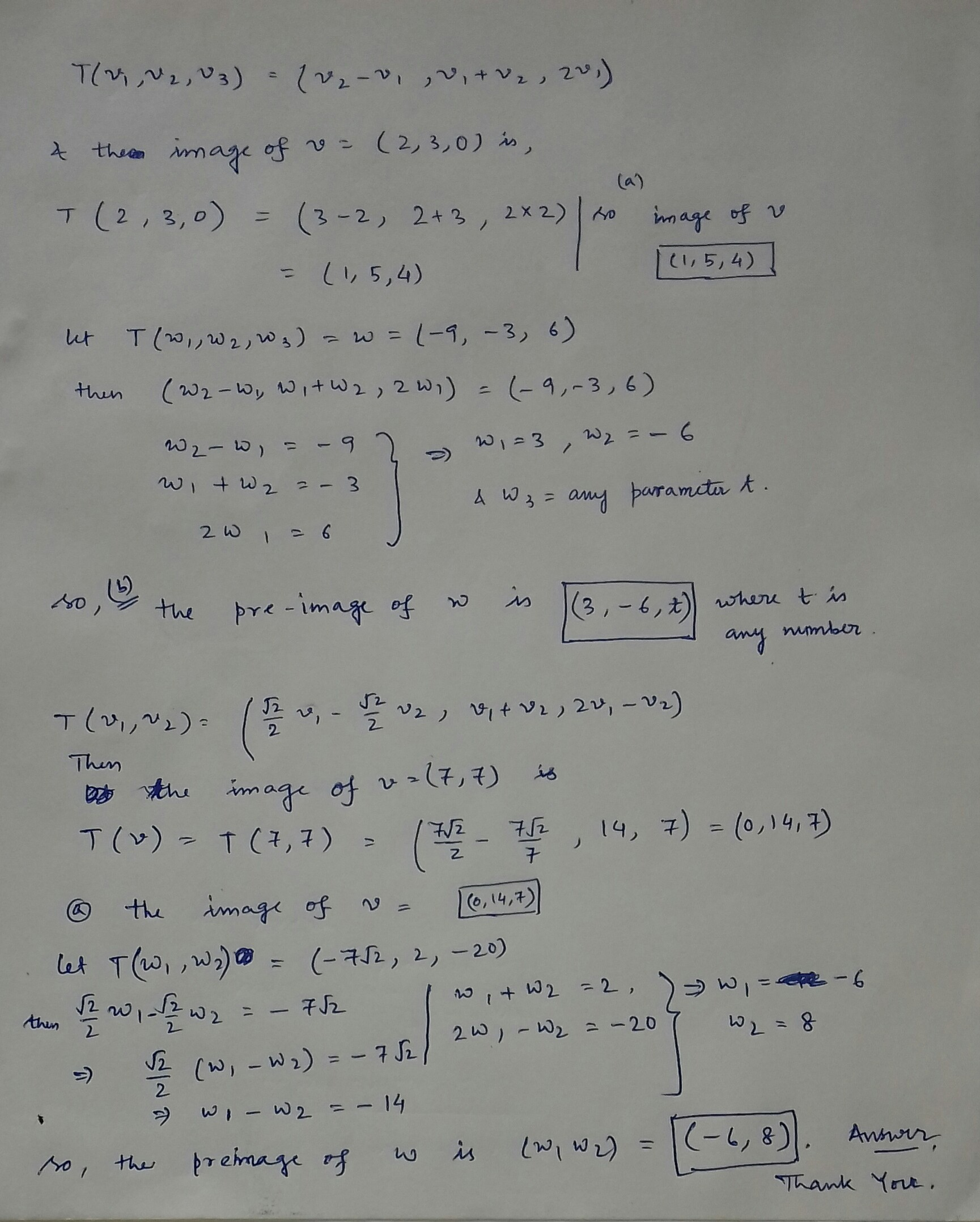If you have any doubt, please comment and I will try my best to explore this to you. Thank you.

Know the answer?
Your Answer:

#### Post as a guest

Your Name:

What's your source?

#### Earn Coin

Coins can be redeemed for fabulous gifts.

Not the answer you're looking for? Ask your own homework help question. Our experts will answer your question WITHIN MINUTES for Free.
Similar Homework Help Questions
• ### Use the function to find the image of V and the preimage of W. T(V1, V2,...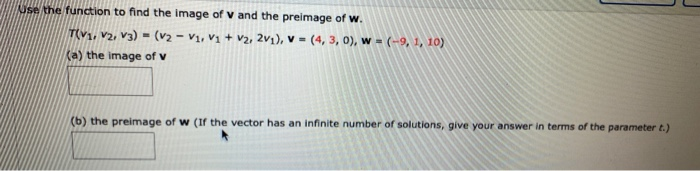Use the function to find the image of V and the preimage of W. T(V1, V2, V3) = (v2 - V1, V1 + V2, 2v1), V = (4,3,0), w = (-9, 1, 10) (a) the image of v (b) the preimage of w (If the vector has an infinite number of solutions, give your answer in terms of the parameter 1.)

• ### Use the function to find the image of v and the preimage of w. TV1, V2,...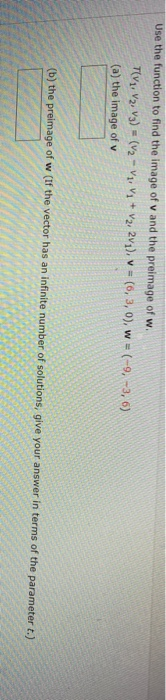Use the function to find the image of v and the preimage of w. TV1, V2, V3) = (V2 - Vz, V; + V2, 2v1), v = (6,3,0), w = (-9, -3, 6) (a) the image of v (b) the preimage of w (If the vector has an infinite number of solutions, give your answer in terms of the parameter t.)

• ### linear algebra Use the function to find the image of v and the preimage of w....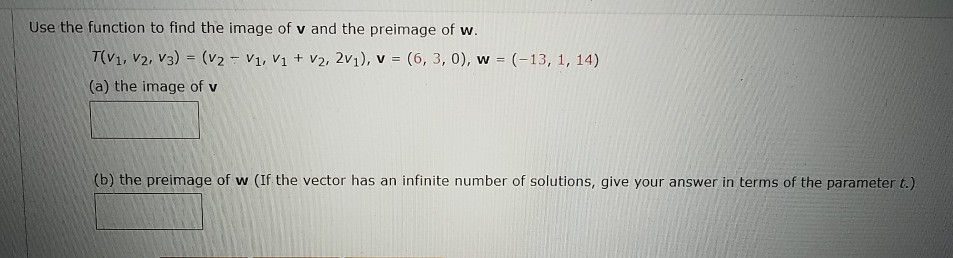linear algebra Use the function to find the image of v and the preimage of w. T(V1, V2, V3) = (v2 - V1, V1 + V2, 2v1), v = (6,3,0), w = (-13, 1, 14) (a) the image of v (b) the preimage of w (If the vector has an infinite number of solutions, give your answer in terms of the parameter t.)

• ### -/2 POINTS LARLINALG8 6.1.001. Use the function to find the image of v and the preimage...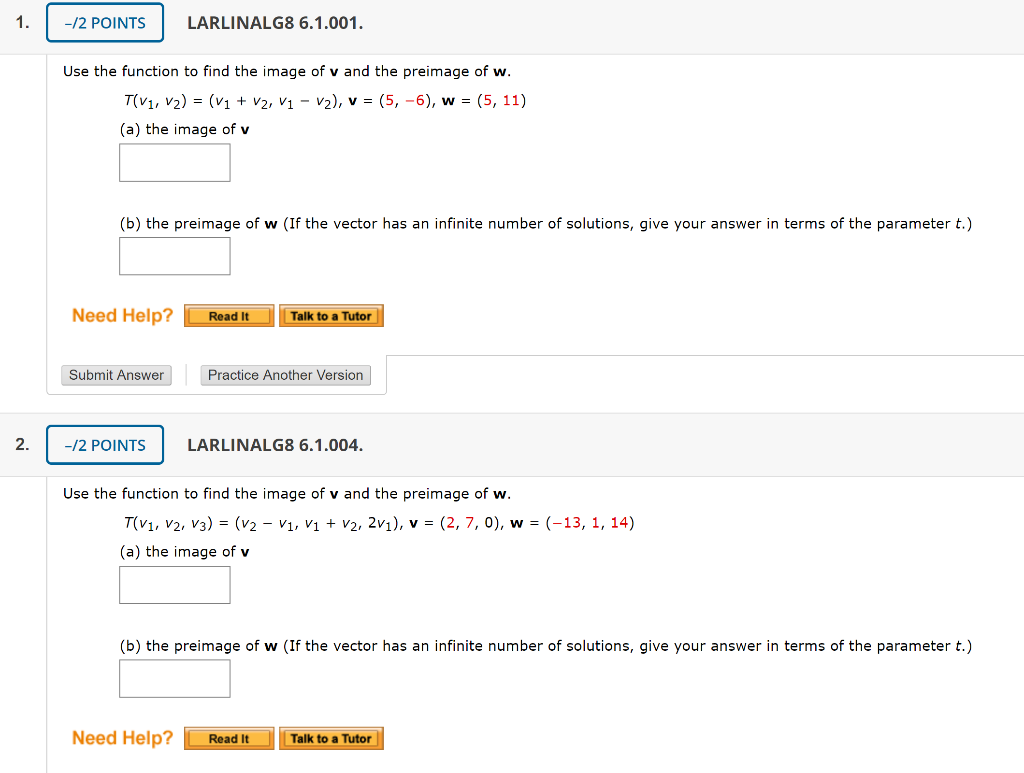-/2 POINTS LARLINALG8 6.1.001. Use the function to find the image of v and the preimage of w. T(V1, V2) = (v1 + V2, V1 - v2), v = (5, -6), w = (5, 11) (a) the image of v (b) the preimage of w (If the vector has an infinite number of solutions, give your answer in terms of the parameter t.) Need Help? Read It Talk to a Tutor Submit Answer Practice Another Version -/2 POINTS LARLINALG8 6.1.004....

• ### 0.5/1 POINTS PREVIOUS ANSWERS LARLINALG8 6.1.001. MY NOTES ASK YOUR TEACHER Use the function to find...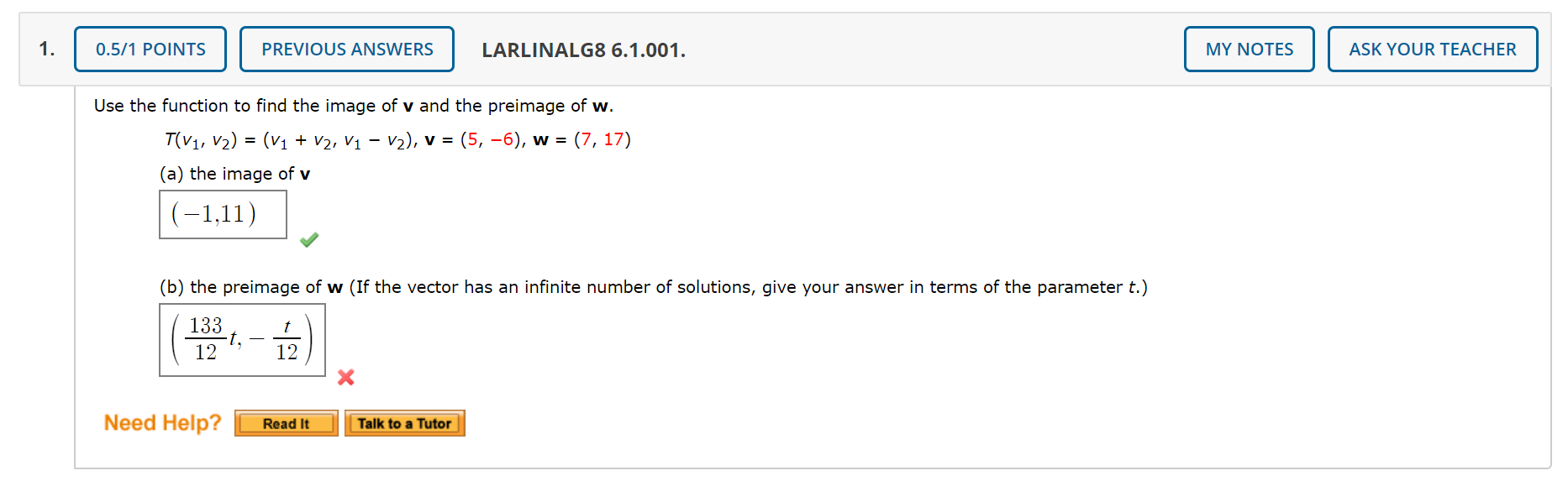0.5/1 POINTS PREVIOUS ANSWERS LARLINALG8 6.1.001. MY NOTES ASK YOUR TEACHER Use the function to find the image of v and the preimage of w. T(V1, V2) = (V1 + V2, V1 – V2), v = (5, -6), w = (7, 17) (a) the image of v |(-1,11) (b) the preimage of w (If the vector has an infinite number of solutions, give your answer in terms of the parameter t.) | 133 ( 1321,- Need Help? Read It Talk...

• ### Let {v1, v2,v3} be a linearly independent set in R^n and let v = -αv3 +v1,w...

Let {v1, v2,v3} be a linearly independent set in R^n and let v = -αv3 +v1,w = v2 - αv1, u= v3-αv2 where αER, find all the values of α, where v, w, u are linearly dependent. do not use matrices.

• ### Please use nodal analysis to find V1, V2, and V3 Given the circuit which will be...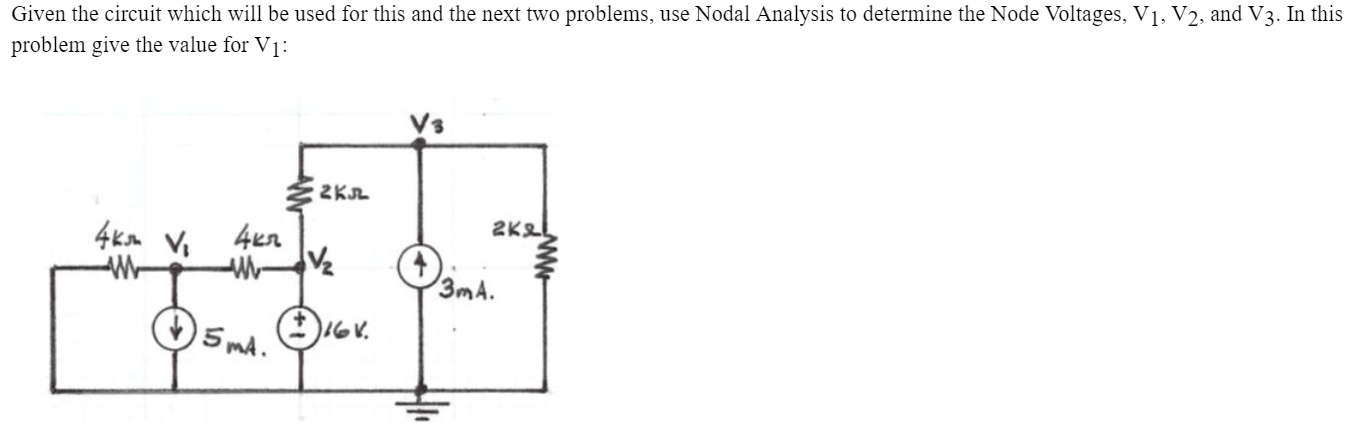Please use nodal analysis to find V1, V2, and V3 Given the circuit which will be used for this and the next two problems, use Nodal Analysis to determine the Node Voltages, V1, V2, and V3. In this problem give the value for V1: 2KR 4ksh Vi 4kr 2KL va 3mA. 15mA. Gr.

• ### 7. Let T : V → W be a linear transformation, and let v1,v2,...,vn be vectors...

7. Let T : V → W be a linear transformation, and let v1,v2,...,vn be vectors in V. Suppose that T (v1), T (v2), . . . , T (vn) are linearly independent. Show that v1, v2, . . . , vn are linearly independent.

• ### 300) V and v2 (t) 20 cos(wt 45°) V, find v(t) v1 (t)2(t) If v1(t) =...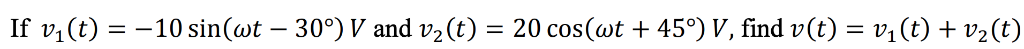300) V and v2 (t) 20 cos(wt 45°) V, find v(t) v1 (t)2(t) If v1(t) = -10 sin(wt _ -

• ### suppose that s=(v1,v2,......vm) is a finite set of linearly independent vectors in V, and w ∈ V some other vector. Let T= S ∪ (W). Prove that T is not linearly independent if and only if w∈ span(s).

suppose that s=(v1,v2,......vm) is a finite set of linearly independent vectors in V, and w ∈ V some other vector. Let T= S ∪ (W). Prove that T is not linearly independent if and only if w∈ span(s).